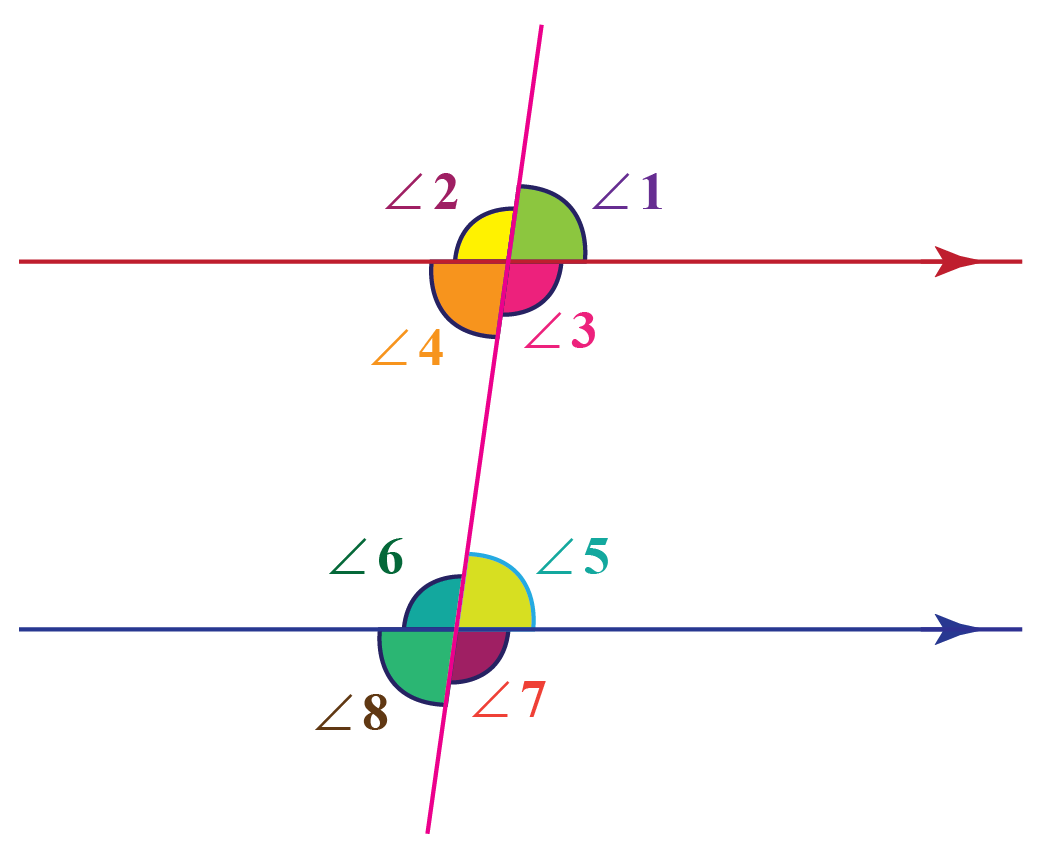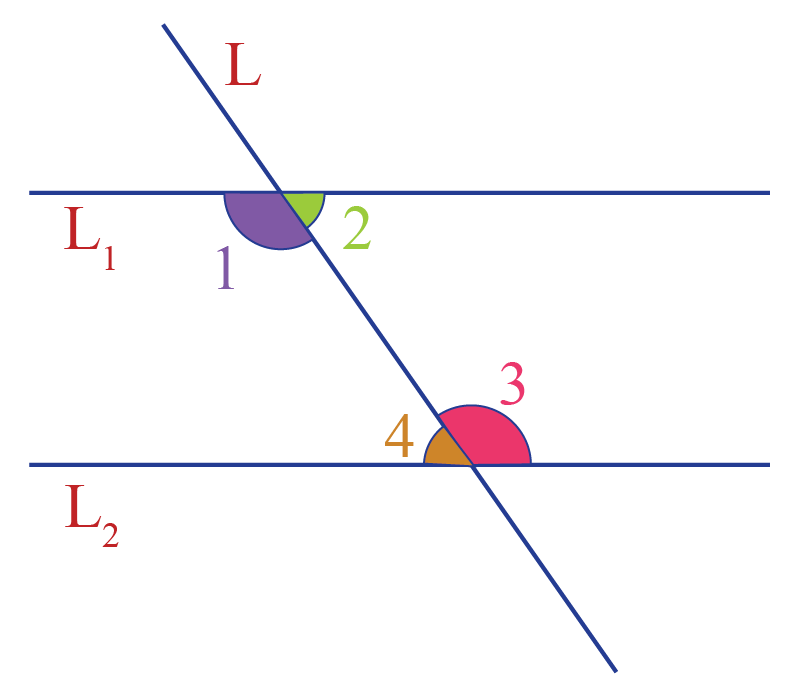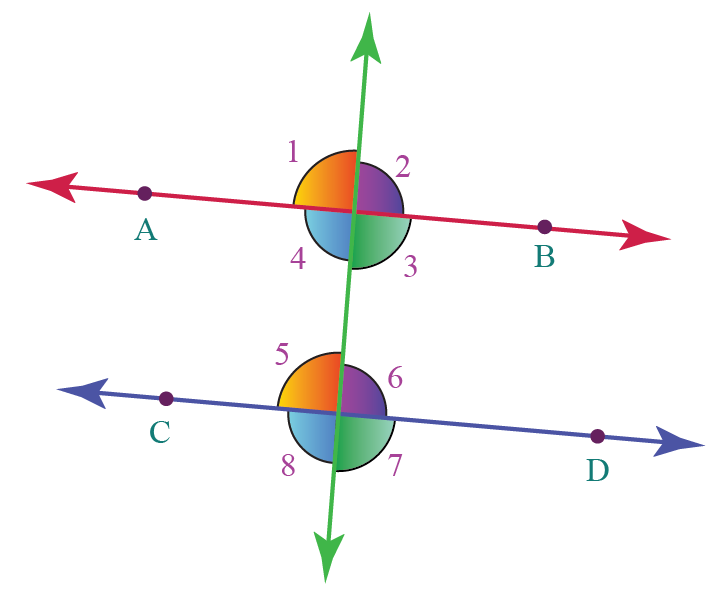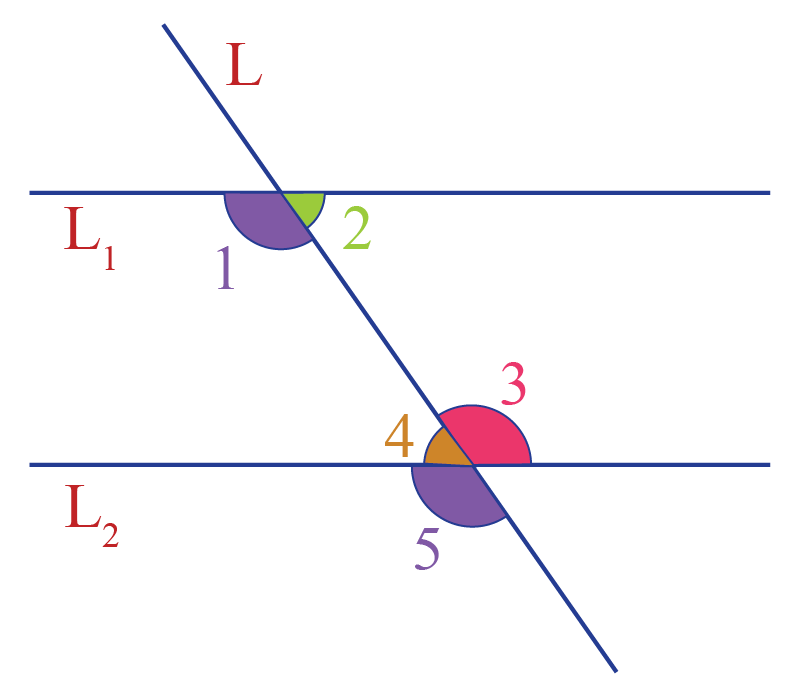# Consecutive Interior Angles

Consecutive Interior Angles

In this mini-lesson, we will explore the world of consecutive interior angles by learning the meaning of consecutive interior angles.

We will also solve some example problems on consecutive interior angles by using the consecutive interior angles theorem.

Let's begin!

Look at the figure below. Can you observe two parallel lines?

When two parallel lines are intersected by a transversal, 8 angles are formed.These 8 angles are classified into four types:

We will study more about "Consecutive Interior Angles" here.

## Lesson PLan

 1 What Is the Meaning of Consecutive Interior Angles? 2 Important Notes on Consecutive Interior Angles 3 Solved Examples on Consecutive Interior Angles 4 Challenging Questions on Consecutive Interior Angles 5 Interactive Questions on Consecutive Interior Angles

## What Is the Meaning of Consecutive Interior Angles?

### Consecutive Interior Angles Definition

Consecutive interior angles are the pair of non-adjacent interior angles that lie on the same side of the transversal.

Consecutive interior angles:

• have different vertices
• lie between two lines
• and are on the same side of the transversal

"Consecutive interior angles" are also known as "co-interior angles" or "same-side interior angles."In the above figure, $$L_1$$ and $$L_2$$ are parallel and $$L$$ is the transversal.

By the consecutive interior angles definition,

Here, the pairs of consecutive interior angles in the above figure are:

• 1 and 4
• 2 and 3

## What Are the Types of Intersection Angles?

When a transversal crosses a pair of parallel lines, you end up with 4 types of intersection angles.If you want to learn about each type of angles in detail, click on the "+" symbol that is against each heading.

## 1. Corresponding Angles

Corresponding angles are angles that:

• have different vertices
• lie on the same side of the transversal and lie above or below the lines
• are always equal

When a transversal intersects two parallel lines, the corresponding angles formed are always equal.

In the above figure, $${\angle 1}$$ & $${\angle 5}$$, $${\angle 2}$$ & $${\angle 6}$$, $${\angle 4}$$ & $${\angle 8}$$, $${\angle 3}$$ & $${\angle 7}$$ are all pairs of corresponding angles.

## 2. Alternate Interior Angles

Alternate interior angles are angles that:

• have different vertices
• lie on the alternate sides of the transversal
• lie between the interior of the two lines

When a transversal intersects two parallel lines, the alternate interior angles formed are always equal.

Here,$${\angle 4}$$ & $${\angle 6}$$ and $${\angle 3}$$ & $${\angle 5}$$ are pairs of alternate interior angles.

## 3. Alternate Exterior Angles

Alternate exterior angles are those angles that:

• have different vertices
• lie on the alternate sides of the transversal
• are exterior to the lines

When a transversal intersects two parallel lines, alternate exterior angles formed are always equal.

Here,$${\angle 1}$$ & $${\angle 7}$$ and $${\angle 2}$$ & $${\angle 8}$$ are pairs of alternate exterior angles.

## 4. Consecutive interior Angles

Consecutive interior angles are those angles that:

• have different vertices
• lie between two lines
• and are on the same side of the transversal

When a transversal intersects two parallel lines, the consecutive interior angles are always supplementary.

Here,$${\angle 4}$$ & $${\angle 5}$$ and $${\angle 3}$$ & $${\angle 6}$$ are pairs of consecutive interior angles.

## Consecutive Interior Angle Theorem

The relation between the consecutive interior angles is determined by the consecutive interior angle theorem.

The "consecutive interior angle theorem" states that if a transversal intersects two parallel lines, each pair of consecutive interior angles are supplementary (their sum is 180$$^\circ$$).

Conversely, if a transversal intersects two lines such that a pair of consecutive interior angles are supplementary, then the two lines are parallel.

Here is the proof of this theorem and its converse.

### Consecutive Interior Angle Theorem - Proof

Look at the following figure.We know that the two lines are parallel, thus we have:

\begin{align} \angle 1& = \angle 5 \;\;\;\text{ (corresponding angles)} \\[0.3cm]\angle 5 + \angle4& = 180^\circ \;\text{ (linear pair)}\end{align}

From the above two equations, $\angle 1 + \angle4 = 180^\circ$

Similarly, we can show that $\angle 2 + \angle 3 = 180^\circ$

### Converse of Consecutive Interior Angle Theorem - Proof

Let us assume that

\begin{align}\angle 1 + \angle4 &= 180^\circ & \rightarrow (1) \end{align}

Since $$\angle 5$$ and $$\angle 4$$ forms linear pair,

\begin{align}\angle 5 + \angle4 &= 180^\circ & \rightarrow (2) \end{align}

From (1) and (2),

$\angle 1 = \angle 5$

Thus, a pair of corresponding angles is equal, which can only happen if the two lines are parallel.

Hence, the converse of consecutive interior angle theorem is proved.

Would you like to observe visually how the consecutive interior angles are supplementary?

Here is an illustration for you to try.

Click on "Go" to see how the "Consecutive Interior Angles Theorem" is true.

More Important Topics
Numbers
Algebra
Geometry
Measurement
Money
Data
Trigonometry
Calculus